# Introduction to Deep Learning: What Is Deep Learning? "To explain" What is Deep Learning? "In an easy-to-understand way even for beginners.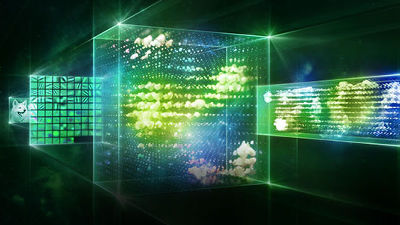Movie which briefly explains "Deep Learning" which is utilized in fields such as image recognition, language translation, artificial intelligence etc.Introduction to Deep Learning: What Is Deep Learning?MATLAB publishes it on YouTube. In the movie, the beginnings of deep learning and concrete practical examples are cited, and it is content that can be imagined about deep learning technology even by novice beginners somehow.

Introduction to Deep Learning: What Is Deep Learning? - YouTube

"What is deep learning which attracts great attention in recent years?" Mr. Shamal Patel of MathWorks said. It is said that there are reasonable reasons for deep learning to draw attention.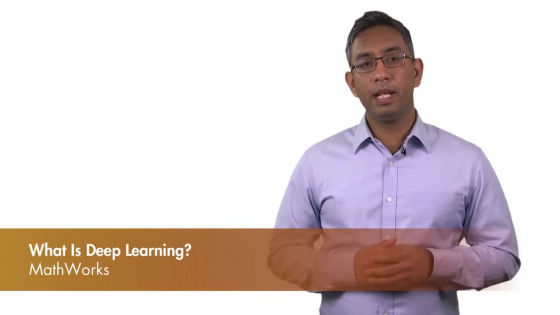Mr. Patel seems to explain three points in the movie, "What is deep learning?" "How it actually is used" "How can I start learning about deep learning"?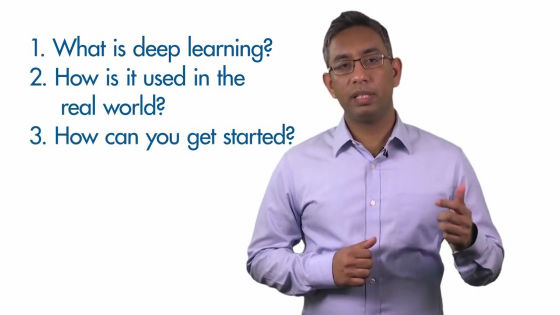What is deep learning?Machine learningOne of the features is that you can learn features and tasks directly from the data. Data consists mainly of images, texts and sounds.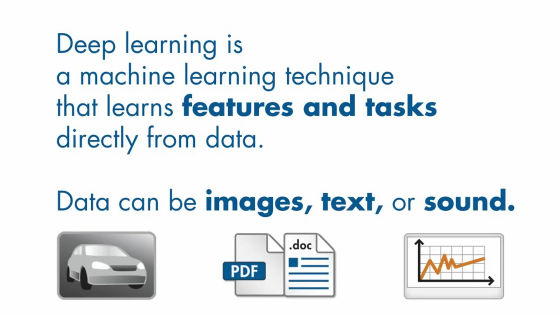In deep learning it is often said that "End to End" learning is possible. I will explain the meaning by taking image recognition as an example.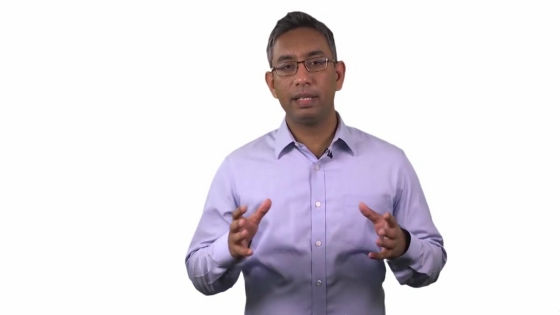Consider sorting an image into categories such as cars, trucks, and boats. Let's start by preparing images of labeled images and training data.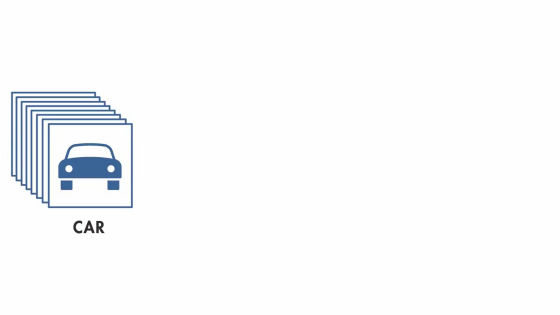The label matches the output required by the task and it is necessary to teach features of the object. The deep learning algorithm learns which category to classify by reading the features from the image image. In this way, we call deep learning "end-to-end learning" from the features that can be learned directly from data such as images.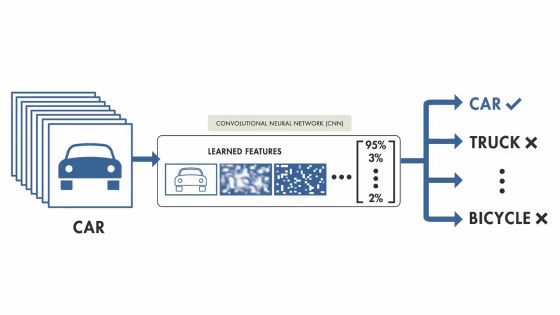Another example of deep learning is a robot.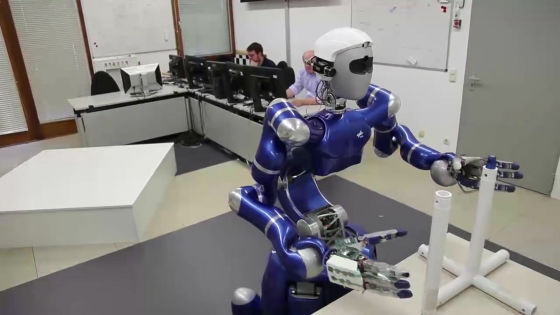The robot is to learn the movement of the object directly using the arm, you can learn how it handled How things. In both examples of image classification and robot arm, image recognition is performed using deep learning.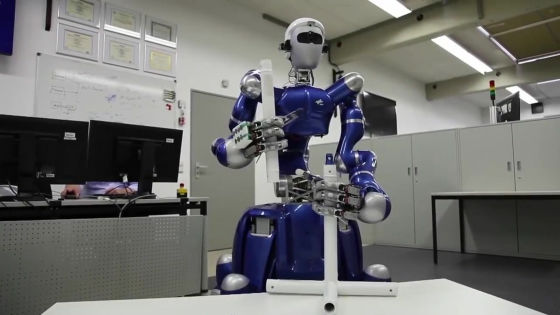Deep learning was originally used for sorting work at the post office. It is the beginning of deep learning that was used for reading and sorting zip codes handwritten in letters in the 1990's.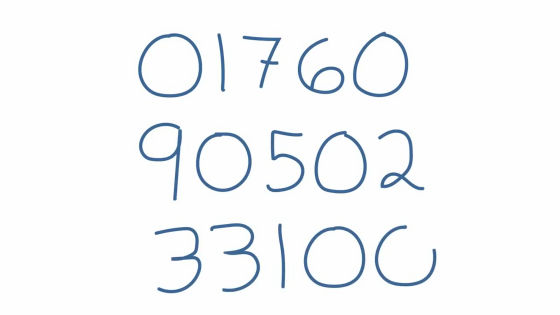However, it has been five years since deep learning technology increased in importance at a stretch. There are three elements to that.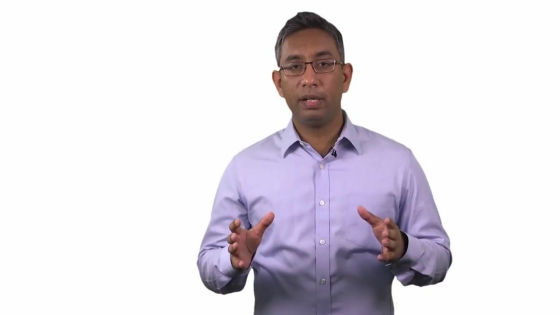First, the deep learning method reached a level that is lower than the error rate for humans in image classification.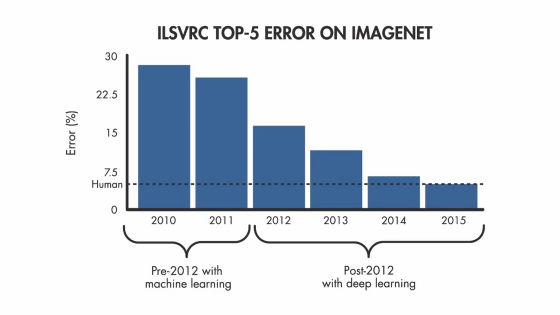Secondly, by improving the performance of the graphic board, the computing capacity has dramatically improved, so that deep network processing can be done in realistic time. The third is that we can now access a large amount of labeled data necessary for deep learning.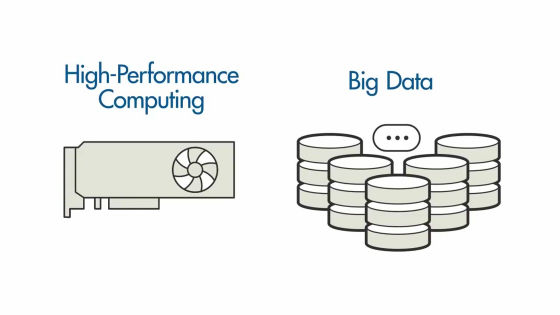Most deep learning methods use the "deep neural network" structure. This is because it treats deep learning and deep neural networks like synonyms.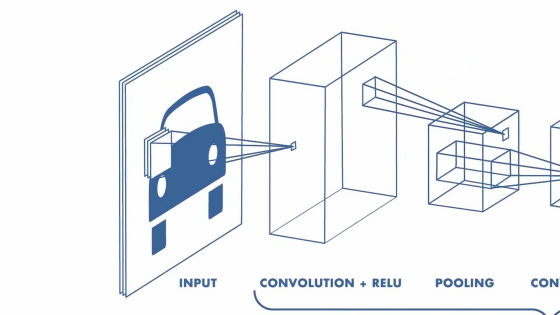The most common deep neural network is "Convolutional Neural Network (Convolution neural networkIn a network known as "CNN" is called. CNN is especially known for being suitable for handling image data.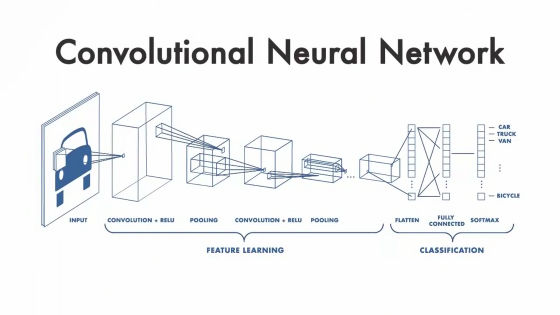The term "deep" usually means that there are a large number of "hidden networks" in the neural network. In classical neural networks there were only 2 or 3 hidden layers, but recent deepler learning uses 150 hidden layers.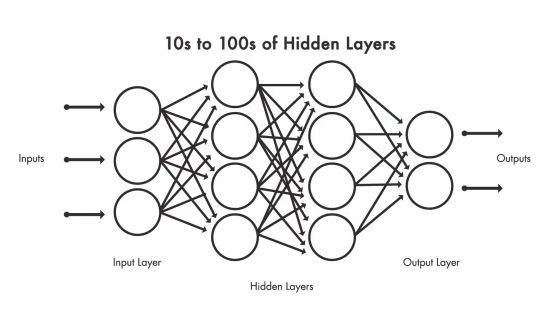"I should have understood the concept that will become the key of deep learning so far so I will explain how deep learning technology is actually used."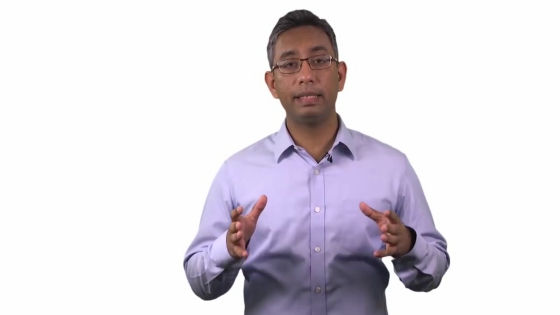This is image recognition technology used in MATLAB. When you display the phone on the desk with a camera, it displays with probability what it is.When you view a notebook PC, it is recognized as "laptop" "notebook". By deep learning, the computer judges "the thing is what?" By matching the characteristics of the subject with the read data.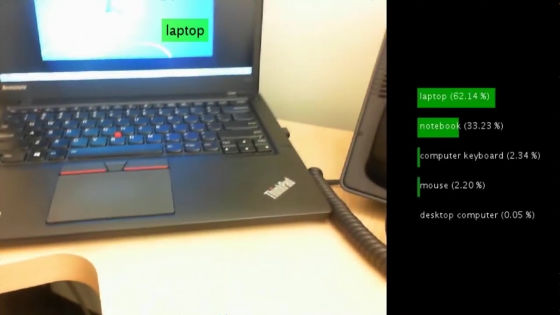This is an example that recognizes from the image of the in-vehicle camera of the running automobile that the object in front is "signpost"."What a deep learning?" Spoke at the beginning movie but we described "actually how they are used" for the, for "How I once you start to learn about deep learning by doing" in the third one, "Mathworks.com/deep-learningI wanted you to see, the movie was concluded.in Software,   Video, Posted by darkhorse_log